# Volume Calculator

By Hanna Pamuła, PhD candidate, Piotr Małek and Mateusz Mucha
Last updated: Aug 09, 2021

The volume calculator will calculate the volume of some of the most common three-dimensional solids. Before we go into how to calculate volume, you must know the definition of volume. Volume differs from the area, which is the amount of space taken up in a two-dimensional figure. So you might be confused as to how to find the volume of a rectangle versus how to find the volume of a box. The calculator will assist in calculating the volume of a sphere, cylinder, cube, cone, and rectangular solids.

## What is volume? — Volume definition

Volume is the amount of space that an object or substance occupies. Generally, the volume of a container is understood as its capacity — not the amount of space the container itself displaces. Cubic meter (m3) is an SI unit for volume.

However, the term volume may also refer to many other things, such as

• the degree of loudness or the intensity of a sound (you may want to check our noise pollution calculator or dB calculator)
• the number or amount of something (usually large quantity)
• formal word for a book or one in a set of related books.

## Volume units and conversion table

Popular units of volume are:

1. Metric volume units
• Cubic centimeters (cm³)
• Cubic meters (m³)
• Liters (l, L)
• Milliliters (ml, mL)
2. US Standard, UK
• Fluid ounce (fl oz)
• Cubic inch (cu in)
• Cubic foot (cu ft)
• Cups
• Pints (pt)
• Quarts (qt)
• Gallons (gal)

If you need to convert the units of volume, you can use our great volume converter. Another useful tool is our grams to cups calculator, which can help if you want to use a food recipe from a different country. Note that it's not a simple conversion, but change from weight (grams) to volume unit (cups) — that's why you need to know the ingredient type (or more specifically, its density).

Also, you can have a look at this neat volume unit conversion table to find out the conversion factor in a blink of an eye:

cubic inches cubic feet cubic yards us liquid gallons us dry gallons imp liquid gallons barrels (oil) cups fluid ounces (UK) fluid ounces (US) pints (UK)
cubic meter 6.1 104 35.3 1.308 264.2 227 220 6.29 4227 3.52 104 3.38 104 1760
cubic decimeter 61.02 0.035 1.3 10-3 0.264 0.227 0.22 0.006 4.23 35.2 33.8 1.76
cubic centimeter 0.061 3.5 10-5 1.3 10-6 2.64 10-4 2.27 10-4 2.2 10-4 6.29 10-6 4.2 10-3 3.5 10-2 3.34 10-2 1.76 103
cubic millimeter 6.1 10-5 3.5 10-8 1.31 10-9 2.64 10-7 2.27 10-7 2.2 10-7 6.3 10-9 4.2 10-6 3.5 10-5 3.4 10-5 1.76 10-6
hectoliters 6.1 103 3.53 0.13 26.4 22.7 22 0.63 423 3.5 103 3381 176
liters 61 3.5 10-2 1.3 10-3 0.26 0.23 0.22 6.3 10-3 4.2 35.2 33.8 1.76
centiliters 0.61 3.5 10-4 1.3 10-5 2.6 10-3 2.3 10-3 2.2 10-3 6.3 10-5 4.2 10-2 0.35 0.338 1.76 10-2
milliliters 6.1 10-2 3.5 10-5 1.3 10-6 2.6 10-4 2.3 10-4 2.2 10-4 6.3 10-6 4.2 10-3 3.5 10-2 3.4 10-2 1.76 10-3
cubic inches 1 5.79 10-4 2.1 10-5 4.3 10-3 3.7 10-3 3.6 10-3 10-4 6.9 10-2 0.58 0.55 2.9 10-2
cubic feet 1728 1 0.037 7.48 6.43 6.23 0.18 119.7 997 958 49.8
cubic yards 4.7 104 27 1 202 173.6 168.2 4.8 3232 2.69 104 2.59 104 1345
us liquid gallons 231 0.134 4.95 10-3 1 0.86 0.83 0.024 16 133.2 128 6.7
us dry gallons 268.8 0.156 5.76 10-3 1.16 1 0.97 0.028 18.62 155 148.9 7.75
imp liquid gallons 277.4 0.16 5.9 10-3 1.2 1.03 1 0.029 19.2 160 153.7 8
barrels (oil) 9702 5.61 0.21 42 36.1 35 1 672 5596 5376 279.8
cups 14.4 8.4 10-3 3.1 10-4 6.2 10-2 5.4 10-2 5.2 10-2 1.5 10-3 1 8.3 8 0.4
fluid ounces (UK) 1.73 10-3 3.7 10-5 7.5 10-3 6.45 10-3 6.25 10-3 1.79 10-4 0.12 1 0.96 5 10-2
fluid ounces (US) 1.8 10-3 3.87 10-5 7.8 10-3 6.7 10-3 6.5 10-3 1.89 10-4 0.13 1.04 1 0.052
pints (UK) 34.7 0.02 7.4 10-4 0.15 0.129 0.125 3.57 103 2.4 20 19.2 1

## How to calculate volume? — Volume formulas

There is no simple answer to this question, as it depends on the shape of the object in question. Here are the formulas for some of the most common shapes:

1. Cube = `s³`, where s is the length of the side.

2. Sphere = `(4/3)πr³`, where r is the radius.

3. Cylinder = `πr²h`, where r is the radius and h is the height.

4. Cone = `(1/3)πr²h`, where r is the radius and h is the height.

5. Rectangular solid (volume of a box) = `lwh`, where l is the length, w is the width and h is the height (a simple pool may serve as an example of such shape).

6. Pyramid = `(1/3)Ah` where A is a base area and h is the height. For a pyramid with a regular base, other equation may be used as well: Pyramid = `(n/12) * h * side_length² * cot(π/n)`, where n is a number of sides of the base for a regular polygon.

7. Prism = `πAh`, where A is a base area and h is the height. For a right triangular prism, the equation can be easily derived, as well as for a right rectangular prism, which is apparently the same shape as a box.

Shape Name Formula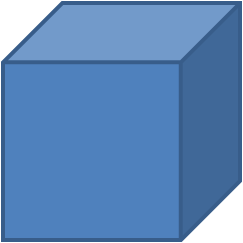Cube V = s³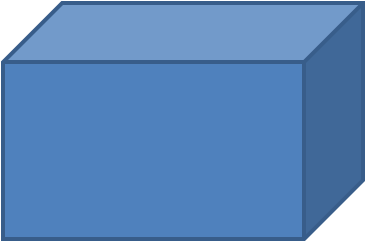Right Rectangular Prism (cuboid, box) V = lwh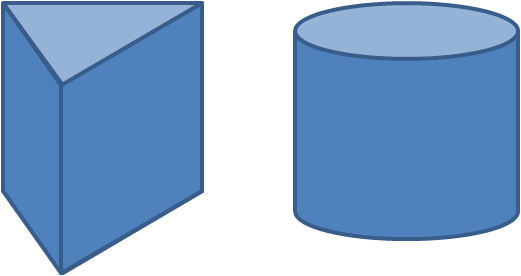Prism or Cylinder V = Ah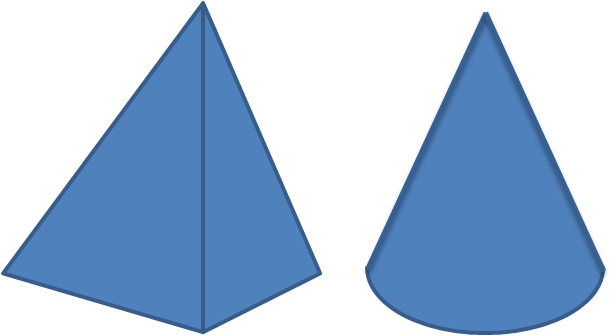Pyramid or Cone V = Ah/3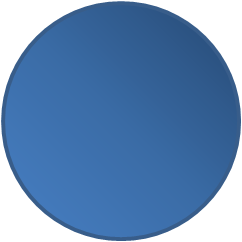Sphere V = 4πr³/3

## Volume calculator and tools dedicated to specific shapes

We've decided to make this volume calculator a simple tool which covers the five most popular 3D shapes. However, not every volume equation and shape type may be implemented here, as it will make the calculator overloaded and unintuitive. So if you're looking for a specific shape, check out the calculators dedicated to volumes of chosen shapes:

## How do I use the volume calculator?

Let's look at the example of how to use this volume calculator:

1. Select the 3D shape type. If you can't find the shape you want to calculate the volume of, choose other special dedicated calculators (you'll find the links above). In this example, let's assume you want to calculate the volume of a cylinder.

2. Choose the right section of the volume calculator. In our case, it's the part titled Cylinder volume.

3. Type given data into proper boxes. Our cylinder has a radius of 1 ft and a height of 3 ft. You can change the units by a simple click on the unit name.

4. Here you go! The volume of a chosen shape is displayed. In our case, it's 9.42478 cu ft.

If you want to check how much that is in US barrels unit, just click on the unit name and choose barrels from the drop-down list. Our cylinder has a capacity of ~2.24 oil barrels.

How to find the volume of objects with different states of matter?

1. SolidFor regular three-dimensional objects, you can easily calculate the volume by taking measurements of its dimensions and applying the appropriate volume equation. If it's an irregular shape, you can try to do the very thing that caused Archimedes to shout the famous word *Eureka*! Probably you heard that story - Archimedes was asked to find out if the Hiero's crown is made from pure gold or just gold-plated - but without bending or destroying it. The idea came to him when he was taking a bath - stepping into a bathtub, he noticed that the water level rose. From this observation, he deduced that volume of water displaced must be equal to the volume of the part of his body he had submerged. Knowing the irregular object volume and its weight, he could calculate the density and compare it with the density of pure gold. Legend says that Archimedes was so excited about this discovery that he popped out of his bathtub and ran naked through the streets of Syracuse.

So, if you want to measure an irregular object's volume, just follow Archimedes footsteps (though you can omit the naked race part):• Take a container bigger than the object you want to measure the volume of. It may be a bucket, a measuring cup, a beaker, or a graduated cylinder. It should have a scale.

• Pour water into the container and read the volume measurement.

• Put the object inside. It should be totally submerged to measure the objects whole volume. Read off the volume. This method won't work if your object dissolves in water.

• The difference between the measurements is the volume of our object.

These measurements are essential in calculating the buoyancy force which are based on Archimedes' principle.

2. LiquidUsually, it's quite easy to measure the volume of a liquid - all you need to have is a graduated measuring vessel of some kind. Choose the one that fits your needs: the amount of liquid and degree of accuracy are the parameters to consider. The containers used in baking a cake (check out awesome calculator for a pancake recipe) will be different to those used in chemistry (e.g. in molar concentration calculations) will be different to ones used for medical purposes (like dose of a medication).3. Gas

We have to use more elaborate methods to measure the volume of a gas. You need to remember that the volume of gas is influenced by temperature and pressure, and that gases expand to fill any container in which they're placed. You can try to measure it:

• Inflate a balloon with the gas you want to measure (e.g., with helium, to lift you up in the air). Then you can use the Archimedes method - put the balloon into the bucket with water and check the volume difference. You'll find detailed instructions on the wikihow page.

• Check out the measures connected to your lung capacity by using a device called spirometer.

• In chemistry, a gas syringe is used to insert or withdraw a volume of a gas from a closed system. This laboratory glassware can also be used to measure the volume of gas evolved from a chemical reaction.

Or calculate:

• Find the volume of the gas, given its density and mass. Use simple `V = m / d` volume equation.

• Calculate the volume of a compressed gas in a cylinder applying ideal gas equation.

## How to find the volume of a rectangle vs volume of a box

You can't calculate a volume of a rectangle, a volume of a circle or a volume of a square because they are 2-dimensional geometric figures. As such, a rectangle does not have a volume (but it does have an area). What you're probably looking for is the volume of a rectangular cuboid (or, in more common terms, you want to find the volume of a box), which is a 3-dimensional object.

To find the volume of a box, simply multiply length, width, and height — and you're good to go! For example, if a box is 5×7×2 cm, then the volume of a box is 70 cubic centimeters. For dimensions that are relatively small whole numbers, calculating volume by hand is easy. For larger or decimal valued numbers, the use of the volume calculator is very efficient.

There are many applications in real life where the volume calculator is useful. One such instance is in road or pavement construction, where slabs of concrete must be built. Generally, concrete slabs are rectangular solids, so the concrete calculator — which is an application of the volume calculator — can be used.

Also, the volume formulas may be helpful if you are a keen gardener or just a happy owner of a house with a yard. Check out our awesome tools such as:

Moreover, you can meet volume in your kitchen or bathroom: any liquid we drink (e.g. bottled water), as well as beauty products or a toothpaste have a volume written on the product packaging (either in milliliters/liters or fluid ounces/gallons).

Another related application, although slightly different, is the concept of surface area. Suppose the entire exterior of a building must be painted. To know how much paint must be purchased, the surface area of the building must be calculated. The convenient to use surface area calculator will calculate this for you.

## FAQ

### How to find volume?

The volume formula depends on the shape of the object. One of the most popular shapes is a rectangular prism, also known as a box, where you can simply multiply length times width times height to find its volume. Another common shape is a cylinder — to find its volume, multiply the height of the cylinder by the area of its base (π × r2). For other 3D shapes, check Omni's Volume Calculator.

### How do I measure volume?

Measuring the volume depends on your object's state of matter. For liquids, you can use a graduated cylinder or burette for the chemistry lab measurements, or a measuring cup & spoon for everyday life purposes. For gases, to roughly measure the volume, you can inflate a balloon and use it to displace the water in a graduate cylinder. A similar method works for solids — put the object into a graduated container and measure the change in reading.

### Is volume squared or cubed?

Volume is "cubed", as it's a three-dimensional measure. Area is a "squared" value, as the area of a shape covers two dimensions. You can remember that volume is a cubed value by recalling a few volume unit names, such as cubic meters, cubic feet or cubic yards.

### How do I calculate volume?

Depending on your object shape, you can use different formulas to calculate volume:

• Cube volume = side3
• Cuboid (rectangular box) volume = length × width × height
• Sphere volume = (4/3) × π × radius3
• Cylinder volume = π × radius2 × height
• Cone volume = (1/3) × π × radius2 × height
• Pyramid volume = (1/3) × base area × height

### What is volume measured in?

A cubic meter is the SI volume unit. However, as it's quite impractical, most often you will encounter volume expressed in:

• Cubic centimeters
• Cubic inches
• Milliliters
• Liters
• Gallons

### How to find volume of a liquid?

Graduated cylinders and Erlenmeyer flasks will work if you need to roughly measure the volume of a liquid. For more precise measurements, you need to use a volumetric pipette and burette. However, if you're baking a cake or prepare a delicious dish and the recipe uses volume units, you can simply use a measuring cup, glass or spoon.

### What is the SI unit for volume?

The cubic meter (m3) is the SI unit of volume. It is derived from the base SI unit of length — the meter. Although the cubic meter is the basic SI unit, other units are used more often: for the metric system milliliters, liters, or cubic centimeters are popular choices, while for the Imperial system you can find volume expressed in pints, gallons, cubic inches, cubic feet or cubic yards.

### Is volume intensive or extensive?

Volume is an extensive property, the same as the amount of substance, mass, energy, or entropy. An extensive property is a measure that depends on the amount of matter. Check out this example: a glass, a barrel, and a pool full of water have different volumes & masses (extensive properties), but water in these three containers will have the same density, refractive index & viscosity (intensive properties).

### What is the difference between surface area and volume?

A volume is a 3D measure, while surface area is two-dimensional. The volume tells us about the cubic space that an object occupies, and the surface area is the sum of all areas forming the 3D shape. Take the cardboard box as example 📦:

• Volume is the amount of space taken up by the box — simply, it's the space available inside the box.
• Surface area is the space occupied by the box's sides, calculated when painting the sides or wrapping the box in paper.

### How to find the volume of an irregular-shaped object?

You can use the fluid displacement method for the irregular shaped solid objects:

1. Fill the container with water and mark the water level.
2. Drop your object inside and mark the level again. Make sure your object isn't soluble in water.
3. For scaled containers, you can just subtract the original volume from the new volume. And that's it, congrats!

But if your original container doesn't have a scale:

1. Take out the object.
2. Fill your container with water to the second mark, pour that water into a graduated cylinder/another measuring vessel.
3. Repeat step 6 for another marked level and subtract the volumes.
4. Pat yourself on the back — you found the volume of an irregular-shaped object!

### What does volume measure?

The volume measures the amount of space taken up by an object in three dimensions. Another closely related term is capacity, which is the volume of the interior of the object. In other words, the capacity describes how much the container can hold (water, gas, etc.).

### What is the volume of the Earth?

The volume of the Earth is approximately equal to 1.08321×1012 km3 (1.08 quadrillion cubic kilometers), or 2.59876×1011 cu mi (259 trillion cubic miles). You can get this result using the sphere volume formula (4/3) × π × radius3 and assuming that the Earth's average radius is 6,371 kilometers (3,958.76 mi).

### How do I calculate surface area to volume ratio?

To calculate the surface area to volume ratio SA:V, you simply divide the surface area by volume. For some chosen shapes:

• SA:V ratio for cube = (6 × side2) / (side3) = 6 / side
• SA:V ratio for sphere = (4 × π × radius2) / ((4/3) × π × radius3)= 3 / radius
• SA:V ratio for cylinder = (2 × π × radius2 + 2 × π × radius × height) / (π × radius2 × height) = 2 × (radius + height) / (radius × height)
Hanna Pamuła, PhD candidate, Piotr Małek and Mateusz Mucha
Shape
Rectangular prism (box)Width (w)
in
Length (l)
in
Height (h)
in
Volume
cu in
Looking for the volume of a partially filled container, like an aquarium? Then make sure to check our great tank volume calculator!
People also viewed…

### Black Hole Collision Calculator

The Black Hole Collision Calculator lets you see the effects of a black hole collision, as well as revealing some of the mysteries of black holes, come on in and enjoy!

### Circle calc: find c, d, a, r

This Circle calc finds c (circumference), d (diameter), a (area), and r (radius) of a circle.

### Korean age

If you're wondering what would your age be from a Korean perspective, use this Korean age calculator to find out.

### Root

The root calculator is sure to become your favorite tool to find the n-th root of a given number.